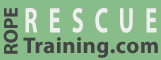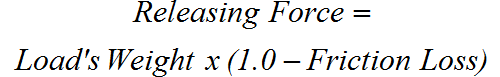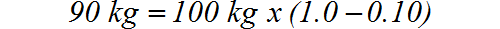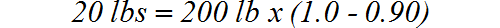# Lowering Friction

## Calculating Friction when Lowering

The formula to calculate friction when lowering a load is:In this illustration a 100-kilogram load is being lowered through a pulley with 10% friction. Using the above formula and substituting 100 kilograms for the weight and 0.1 (i.e., 10%) for the friction loss shows that the hand must hold 90 kilograms. The "missing" 10 kilograms is lost to the pulley's friction.In this case, friction is your friend. The resistance in the pulley consumed 10 pounds of force, reducing the force that you need to hold from 100 kilograms to 90 kilograms. That is much more than a rescuer can hold, but it illustrates the effects of friction.

This next illustration shows a brake bar rack lowering a 200-pound load. Unlike the meager 10% friction imposed by the preceding pulley, this brake bar rack provides 90% friction. Using the same formula, you can see that you only need to hold 20 pounds of the 200-pound load. The rack is absorbing the other 180 pounds.Try It.
Risk Free.